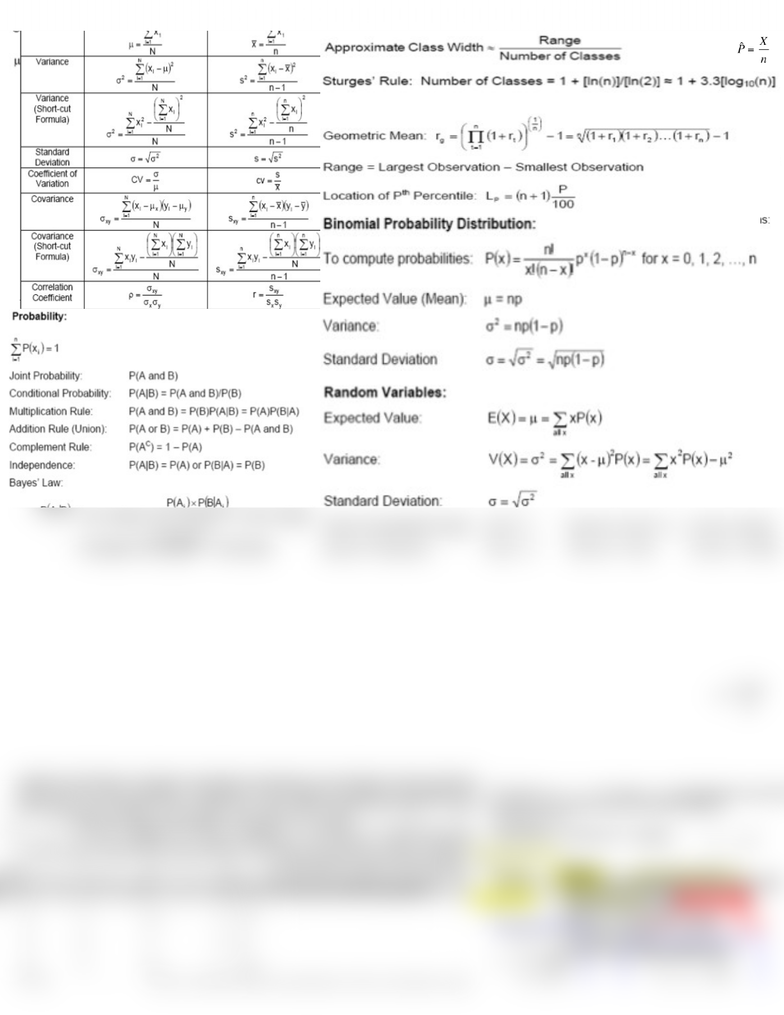# ADMS 2320 Study Guide - Final Guide: Central Limit Theorem, Sampling Distribution, Tachykinin Receptor 1

469 views2 pages
School
Course
Professor1k for
k
1
1
2
Qualities of Estimator: Unbiased- population parameter is an estimator whose expected
value equal to that parameter. Consistent- if the difference between estimator and
parameter grows smaller as the sample size grows larger. Relatively efficient- if there
are two unbiased estimator of parameter, one variance is smaller.
The width of the confidence interval estimate is a function of confidence level,
population SD and sample size. Larger confidence level produce wider confidence
interval; Larger values of SD producer wider CI; increase sample size decreases width of
confidence interval while confidence level remain unchanged (increase cost of obtaining
Confidence Interval Estimator for population mean (used only when SD if known):
Mutually Exclusive Events: If O1 occurs, then O2 cannot occur; O1 and O2 have no common elements
If E1 and E2 are mutually exclusive, then P(E1 and E2) = 0. If independent P(A|B)= P(A) and so on.
Empirical Rule:
If histogram is bell shaped, use Empirical Rule
Approx. 68% of all observation falls within one SD of mean
Approx. 95% falls within two SD of mean, Approx. 99.7 falls within three SD of mean.
The binomial table gives cumulative probabilities for
P(X k), but as we’ve seen in the last example,
P(X = k) = P(X k) – P(X [k1]) Likewise, for probabilities given as P(X k), we have:
P(X k) = 1 – P(X [k1])
σ
μx
z
=
To find P (a < x < b) when x is distributed normally:
-Draw the normal curve for the problem
in terms of x
-Translate x-values to z-values
-Use the Standard Normal Table
Chebyshevs Theorem: any distribution, proportion of observation lying within standard deviation of mean is at least.
The methods of Sampling Plan: Simple Random, Stratified, Cluster. Sampling errors: different samples yield different sampling errors; may be positive or negative; expected sampling error decreases as sample size increases. Nonsampling Error (3 types)= error in data acquisition, nonresponse errors, selection bias (increase in same size will not reduce this error).
Central Limit Theorem: Sampling distribution of mean of random sample from any population is approximately normal for sufficiently large sample size. The larger the sample size, the more closely the sampling distribution of sample mean will resemble normal distribution
Almost all Inference problems involve the same basic steps:
Determining the null and alternate hypotheses, the test statistic and the rejection region; the test statistic is based on the standard deviation of the estimated value; the rejection region is determined by the distribution of the estimate. Only variance varies
Student t-distribution is robust: population is nonnormal, results of t-test and confidence interval estimate are still valid that the population not extremely nonnormal
T-test and estimator of population mean:
Equal variances t-test and estimator of two population means
Steps:
Specify population value of interest
Formulate null/alter
Specify significance level
Construct rejection region
Compute test
Reach decision/conclusion
Unlock document

This preview shows half of the first page of the document.
Unlock all 2 pages and 3 million more documents.